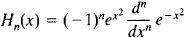# Hermite Polynomials

Also found in: Wikipedia.

## Hermite polynomials

[er′mēt ‚päl·ə′nō·mē·əlz]
(mathematics)
A family of orthogonal polynomials which arise as solutions to Hermite's differential equation, a particular case of the hypergeometric differential equation.
McGraw-Hill Dictionary of Scientific & Technical Terms, 6E, Copyright © 2003 by The McGraw-Hill Companies, Inc.
The following article is from The Great Soviet Encyclopedia (1979). It might be outdated or ideologically biased.

## Hermite Polynomials

a special system of polynomials of successively increasing degree. For n = 0,1, 2,..., the Hermite polynomials Hn (x) are defined by the formulaIn particular, H0 = 1, H1 = 2x, H2 = 4x2 – 2, H3 = 8x3 – 12x, and H4 = 16x4 – 48x2 + 12.

Hermite polynomials are orthogonal on the entire x-axis with respect to the weight function

e –x2

(seeORTHOGONAL POLYNOMIAL). They satisfy the differential equation

y″ – 2xy′ + 2ny = 0

and the recursion formulas

Hn+1 (x ) – 2xHn (x ) + 2nHn-1 (x ) = 0

H′n(x ) – 2nH–1(x ) = 0

Also sometimes called Hermite polynomials are polynomials that differ from those given above by certain factors dependent on n; sometimes

e–x2/2

is used as the weight function. The basic properties of the system were studied by P. L. Chebyshev in 1859 and C. Hermite in 1864.

References in periodicals archive ?
An especially important case occurs for [alpha] = 2, [rho ] = 0, corresponding to the classical Hermite polynomials. Note that the recurrence coefficients for general values of a and [rho ] are not known explicitly, but their asymptotic behavior has been established .
In Section 2 we introduce preliminary results about the n-polyanalytic function spaces and their relationship with the Hermite polynomials. In Section 3 we prove that every Toeplitz operator with bounded horizontal symbol a(z) acting on Fock space is unitary equivalent to a multiplication operator [[gamma].sup.n,[alpha]] (x)I acting on ([L.sub.2]([R.sub.+])).sup.n, where [[gamma].sup.n,a] (x) is a continuous matrix-valued function on (-[infinity], [infinity]).
Polynomial chaos was first introduced by Wiener  where Hermite polynomials were used to model stochastic processes with Gaussian random variables.
In this paper, we use the probabilistic orthonormal Hermite polynomials for [[PSI].sub.i]([xi]) and, accordingly, f([xi]) = (1/[square root of 2[pi]])exp(-[[xi].sup.2]/2).
The wave functions in Schrodinger equation for the well-known potentials have been obtained on the orthogonal polynomials, such as Jacobi, generalized Laguerre, and Hermite polynomials and the energy eigenvalues spectrum can be accessible for each case.
There are only a few explicit examples which include the formula for the exponential generating function of Hermite polynomials  and the Glaisher-Crofton identity [9-11]
These facts, as well as cardinal basis functions perspective, motivated us in  to patch cubic Hermite polynomials together to construct piecewise cubic fuzzy Hermite polynomial and provide an explicit formula in a succinct algorithm to calculate the fuzzy interpolant in cubic case as a new replacement method for [4,10].
Finally, [P.sub.s]([[xi].sup.[alpha]]), [Q.sup.[beta].sub.s] ([[xi].sup.[alpha]]), [R.sup.[beta][gamma].sub.s] ([[xi].sup.[alpha]]), and [S.sup.123.sub.s] ([[xi].sup.[alpha]]) are suitable products of the Hermite polynomials in (4).
Two equivalent versions of the Hermite polynomials may be found in the literature: for n = 0, 1, 2...,
The Hermite analysis functions called [D.sub.n] [20, 21] are a set of orthogonal polynomials obtained from the product of a Gaussian window defined by g and the Hermite polynomials [H.sub.n], where n in this case indicates the order of analysis.
Example 2 Hermite polynomials [{[H.sub.n](t)}.sub.n] forms an orthogonal polynomial system with respect to the linear functional L defined by [Lt.sup.k] = [EX.sup.k], where X is a Gaussian random variable.

Site: Follow: Share:
Open / Close